# Catch up on the latest AI articles# Breaking Through The Barriers Of Computation Time And Memory!

3 main points
✔️ Local-Sensitive-Hashing allows for calculating Attention between necessary elements
✔️ Reversible layers save activation that increases proportionally to the number of layers Memory reduction
✔️ Reduced transformer computation from $O(L^2)$ to $O(L \log L)$.

(Submitted on 13 Jan 2020 (v1), last revised 18 Feb 2020 (this version, v2))

Subjects: Machine Learning (cs.LG); Computation and Language (cs.CL); Machine Learning (stat.ML)code：The images used in this article are from the paper, the introductory slides, or were created based on them.

## Summary

Since the announcement of the Transformer, many huge models that demonstrate high performance in various tasks have been announced, such as BERT, which applies the Attention Layer and stacks up to 24 layers in the Encoder portion of the Transformer, and GPT-2, which stacks up to 48 layers in the Decoder portion. However, because they are so large, they are not suitable for computation. However, the computational cost of such huge models has been increasing rapidly, and research to make models more efficient in a different direction than simply increasing the number of layers to improve performance has also been active. Against this backdrop, Reformer is one of the attempts to make the transformer more efficient.

Reformer is an attempt to deal with the problem that the amount of self-attention in Transformer is $O(n^2)$ and that the memory usage increases rapidly when long sentences are inserted.

## What is the problem with Transformer?

The computational complexity of a transformer increases quadratically with the input sequence. This results in very long computation times and memory usage.

The main cause of the increase in computational complexity is Scaled Dot-Product Self-Attention, a key component of Transformer. To begin with, Scaled Dot-Product Self-Attention calculates Attention using query and key-value pairs. That is, the formula for Scaled Dot-Product Self-Attention uses the query, key, and value ($Q,K,V$) to compute

$$Attention(Q, K, V) = softmax(\frac{QK^T}{\sqrt{d_k}}V)$$

($d_k$ denotes the depth of the query and the value (Q, V). ($d_k$ denotes the depth of the query and the value (Q, V) is then the product of the query and the value (Q, V) is the square of the length of the document ($n$). Therefore, given 2046 tokens as input, the matrix size used in the Attention calculation is 2024*2024, which means that a matrix with about 4.1 million elements must be processed in the Attention calculation.

The Reformer, described in this paper, addresses the Transformer's problem of increasing computational complexity as the input sequence is squared.
The Reformer employs two architectures, Local-Sensitive-Hashing and Reversible Residual layer Reformer employs two architectures, Local-Sensitive-Hashing and Reversible Residual layer, to process long input sequences with less memory.

## Results using Reformer

Experiments comparing Reformer's performance were conducted on two datasets, imagenet64 and enwik8-64k. enwik8-64k is enwik8 divided into 64K tokens. The experimental setup has 3 layers, 1024 dimensions for word embedding and hiddenness ($D_{model}$), 4096 dimensions for the middle layer ($D_{ff}$), and 8 for both the number of heads and batch size.

The right side of Figure 5 in the paper below is a graph of computation time, and it is claimed that the LSH attention of the Reformer proposed in this paper does not increase computation time like the conventional transformer, even when the sentences are long and the number of batches is small. The graphs are shown in the following table.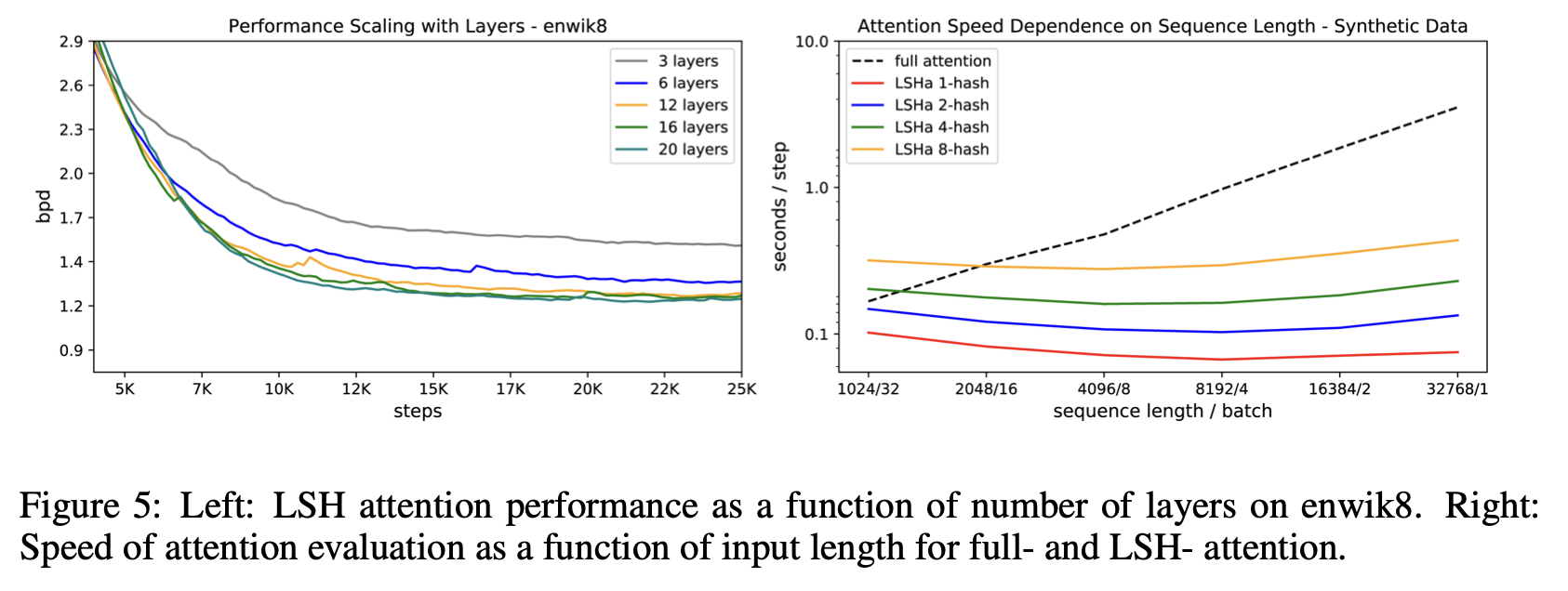Despite the reduction in computation time, the accuracy is almost the same as that of the regular transformer. The accuracy is almost the same as that of a normal transformer (full attention) even with a hash bucket of about 8. It can be seen that even with a hash bucket size of about 8, the performance is almost the same as that of a normal transformer (full attention).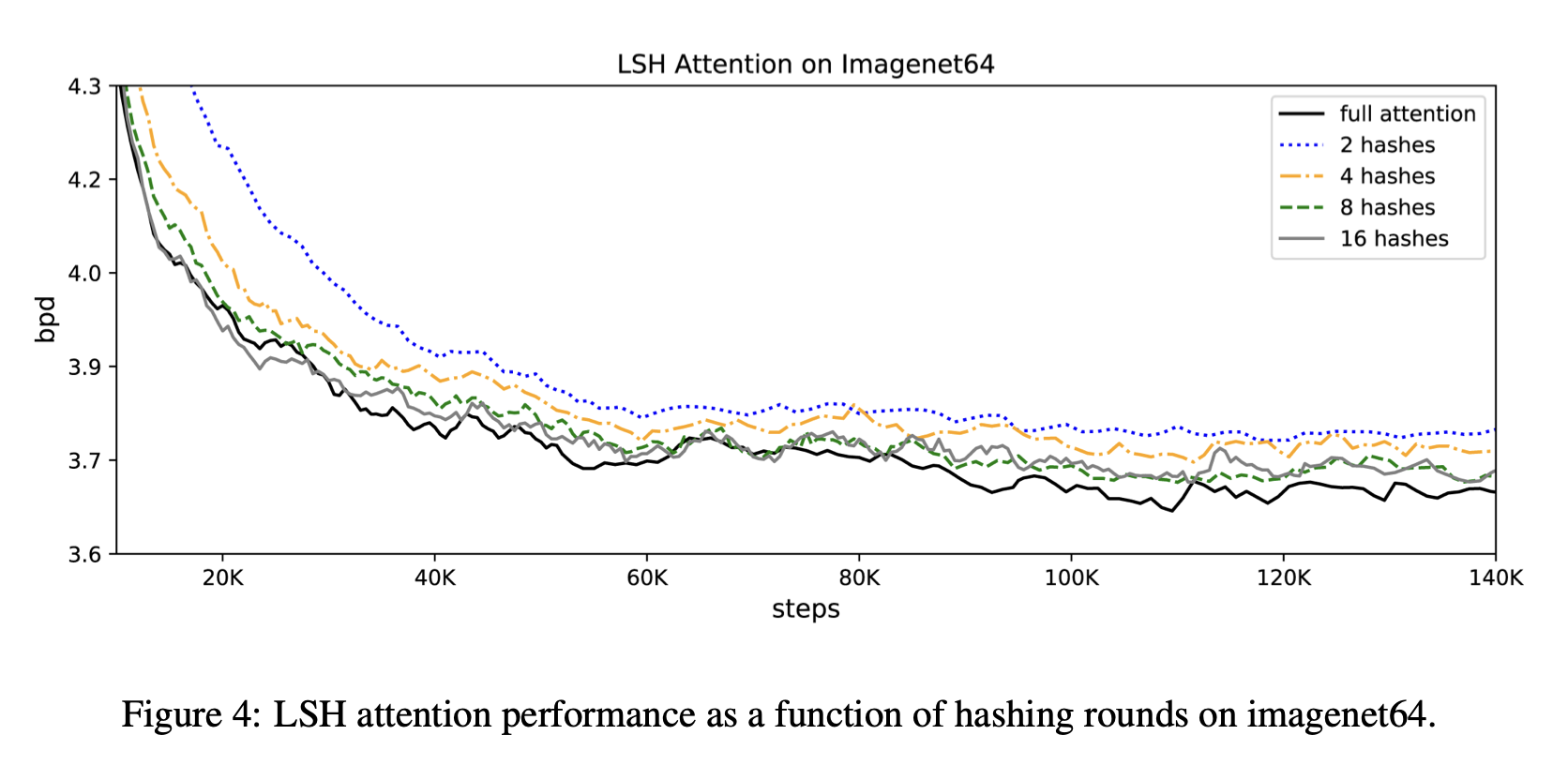In the following, we will see how Reformer has reduced computation time and memory usage.

## Reformer Ingenuity

Reformer uses the following two architectures to significantly reduce memory usage.

• Local-Sensitive-Hashing (LSH)
• Reversible Residual layers

### What is Local-Sensitive-Hashing (LSH)?

The idea of this research is not to calculate all inner products and get keys that are close to the query as in the conventional Attention, but to find a way to get keys that are close to the querywithout calculating all inner products. This is the idea.This is a technique called Local-Sensitive-Hashing (LSH).
To be more specific, using a few mathematical expressions, the conventional Attention can be written as follows.

$$Attention(Q, K, V) = softmax(\frac{QK^T}{\sqrt{d_k}}V)$$

This means that the attention is directed to elements that have little relevance. The argument of this research is that it is inefficient to calculate the entire query, when in fact it should be sufficient to extract only the relevant parts of the query. Therefore, the original idea was to obtain elements (keys) that are similar to the query without calculating Attention for all elements. Therefore, it is not necessary to have all keys, but only a subset of $K$ close to each key $Q_i$ to achieve the goal of $QK^T$. The mathematical expression is as follows.

$$softmax(\frac{q_i K^T}{\sqrt{d_k}}V)$$

We also propose LSH Attention as a mechanism to achieve this.

・LSH Attention

Reformer classifies input using LSH, where similar elements are given the same hash value in order to extract keys that are similar to the query.

Random projection is a method to rotate points scattered in a partitioned 2D plane at a random angle, so that points that are close to each other (similar) have a high probability of moving closer together, while points that are far from each other (dissimilar) will be divided into different partitions. This can be applied to extract only the keys that are close to the query.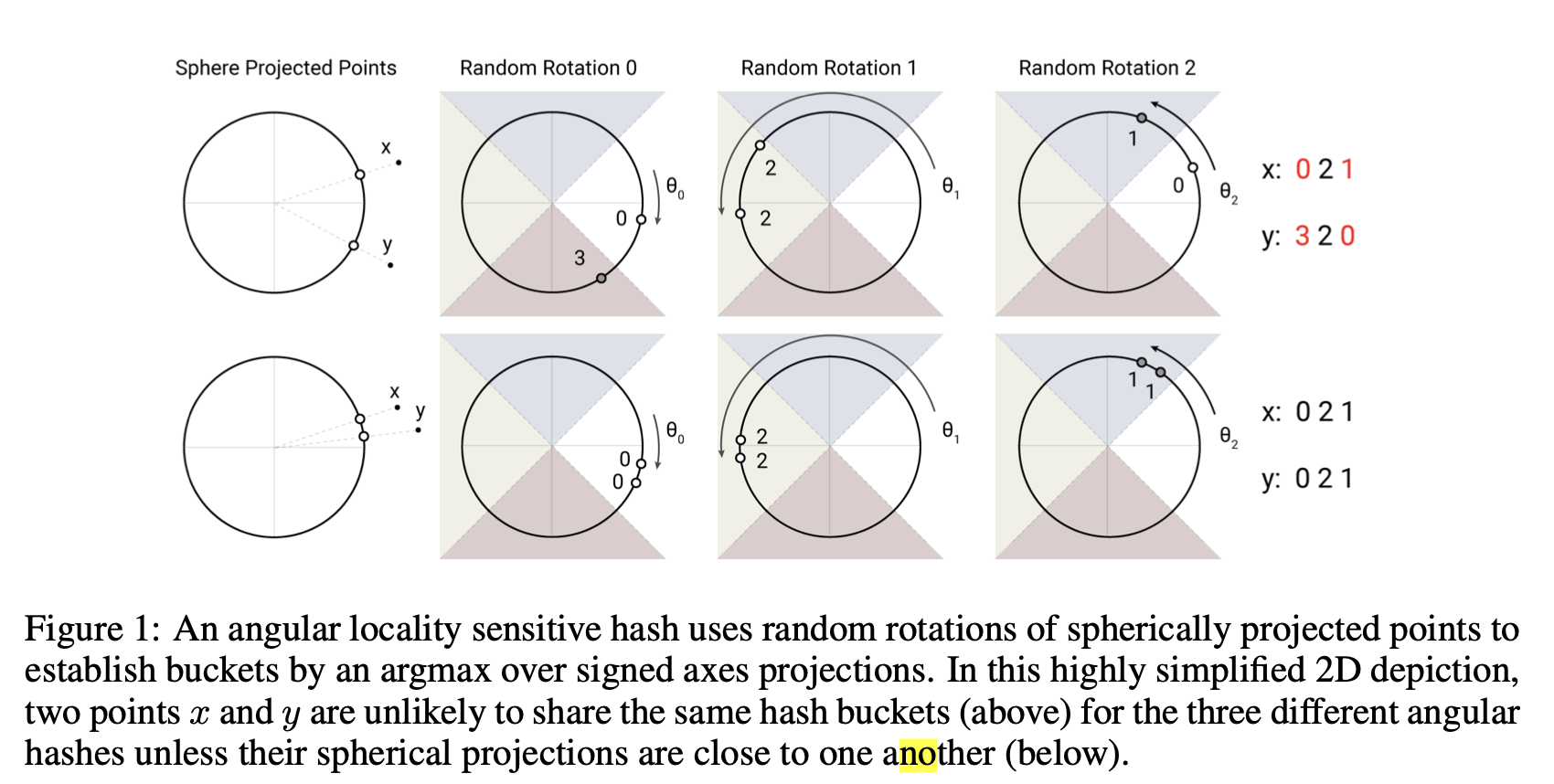Specifically, in the upper figure of Figure 1, the two points are so far apart that they are in the same compartment only once out of three rotations. Conversely, in the lower figure, the two points are closer, so they are in the same compartment for all three random rotations. This partition is called a hash bucket, and the vector is considered to be in the same hash bucket if it is in the same hash bucket after three random rotations. LHS attention is to pay attention only to keys that are in the same bucket as the query $q_i$.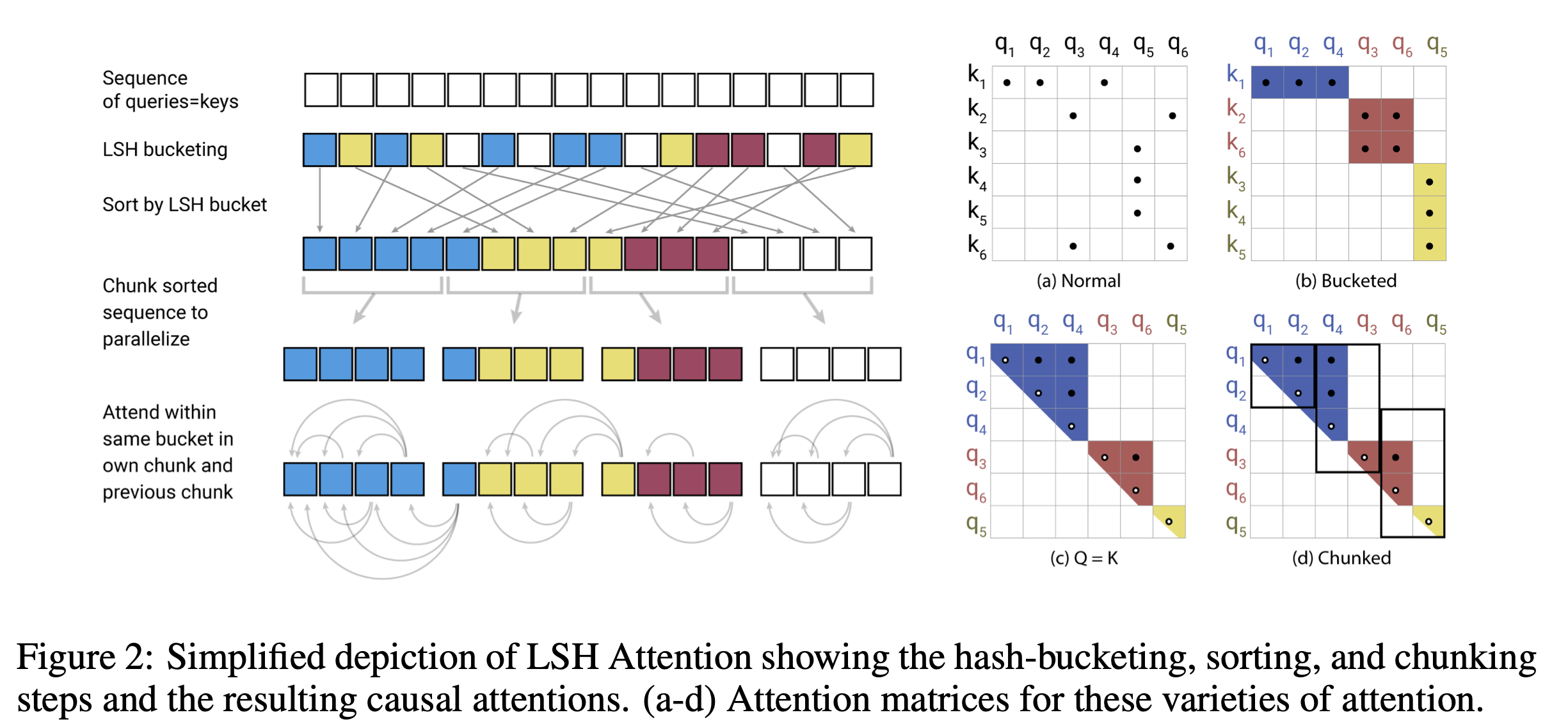More specifically, the behavior of Reformer, as shown in the figure on the left side of Figure 2, is to equate a long sequence of inputs with a query and a key. This is done to avoid the case where there are many queries and no keys in a particular hash. Experiments have proven that there is no problem in making queries and keys the same, as illustrated in Figure 3. (See below.)
After receiving the input, LSH sorts the data into buckets. Sorting is performed on each LSH bucket. Next, the data is divided into chunks of the same length. Attention is calculated for each chunk and the previous chunk, and the keys of interest are extracted.

On the left side of Figure 3, we can see that there is no disadvantage in using the same query and key for both the imagenet64 dataset and Enwiki8.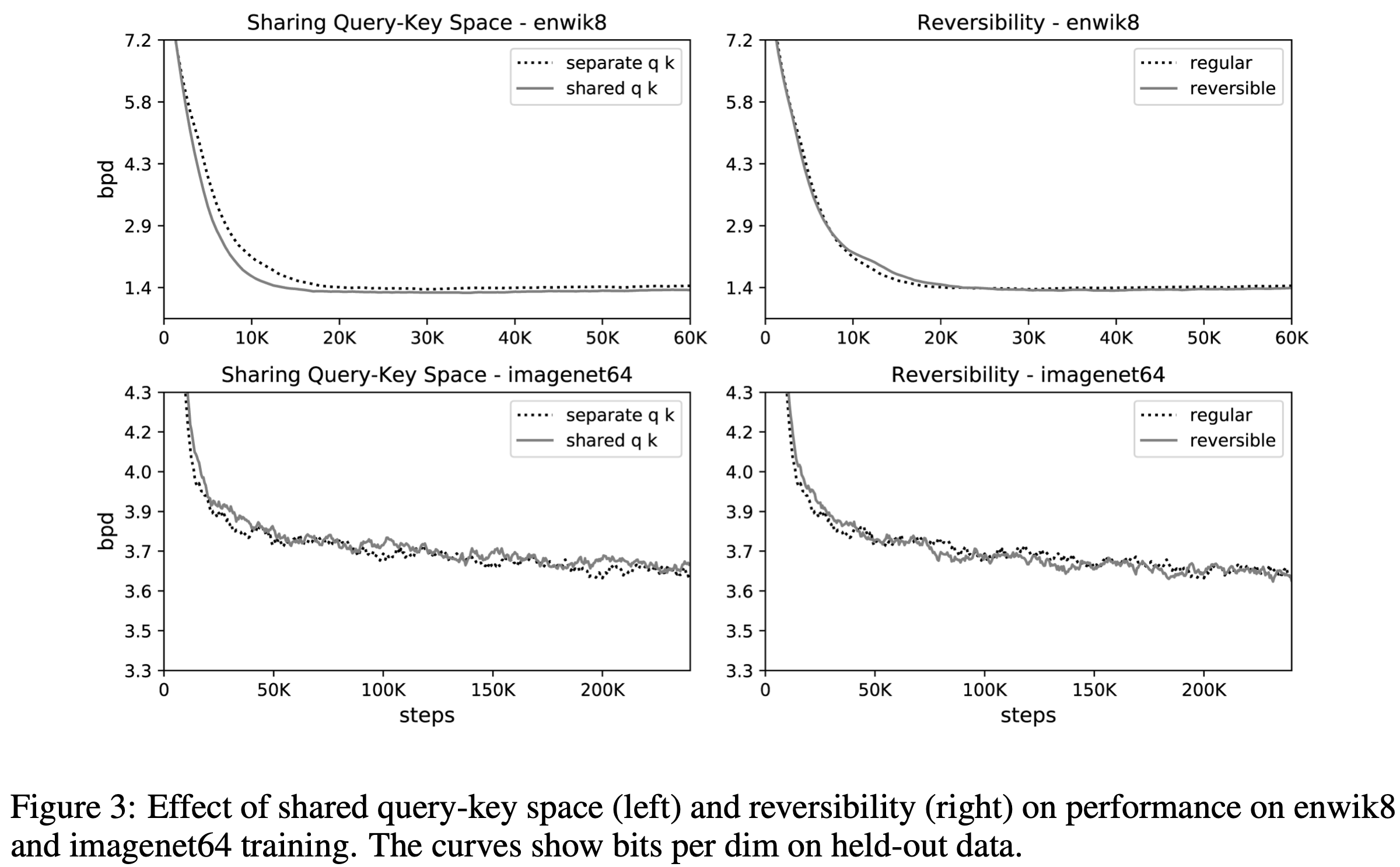### What are Reversible Residual layers?

Another important idea in Reformer is Revirsible Residual layers, which can greatly reduce the computation of Attention by eliminating the need to store intermediate computational states. Specifically, it is usually necessary to store the post-activation values of the activation function in order to perform back-propagation, but the larger the model, such as the BERT and GPT series, the larger the hidden layer dimension and the number of layers, and the more memory is required for storage. Therefore, we attempted to reduce memory usage by using a child that calculates downstream activation function values from upstream layer post-activation activation function values.

Specifically, the Residual Connection is represented by $y = x + F(x)$. This architecture was first adopted in ResNet to avoid the problem of input information not being reflected in the output (loss of information) by adding more layers in deep learning. At the time of ResNet's adoption, the system succeeded in accumulating more efficient learning by adding data directly to the output layer at a specific layer.
A further improvement on the ResNet architecture is the reversible residual network. This network is capable of reversibly computing downstream results to upstream results by splitting the input into two systems.

・RevNet Calculation 1

First, the inputs and outputs are decomposed into two systems $(X_1, X_2)$ and input to two layers (F, G). The resulting computed results are as follows

$$Y_1 = X_1 + F(X_2)$$

$$Y_2 = X_2 + G(X_1)$$

・RevNet Calculation 2

The final output in RevNet is the concatenation of $Y_1$ and $Y_2$.

・Back-propagation in RevNet

Since backpropagation in RevNet can be computed reversibly, it can be shown as follows

$$X_1 = Y_1 - F(X_2)$$

$$X_2 = Y_2 - G(X_1)$$

Although at first glance RevNet may seem to complicate the model, this architecture eliminates the need to store intermediate states during training for back-propagation of output results, as in the past. This is because the values $X_1, X_2$ of the previous activation function needed for back-propagation are computed.
This saves memory by eliminating the need to store all post-activation activation function values that would normally need to be stored. Below is a schematic of the Reversible Residual Network: Backpropagation Without Storing Activations.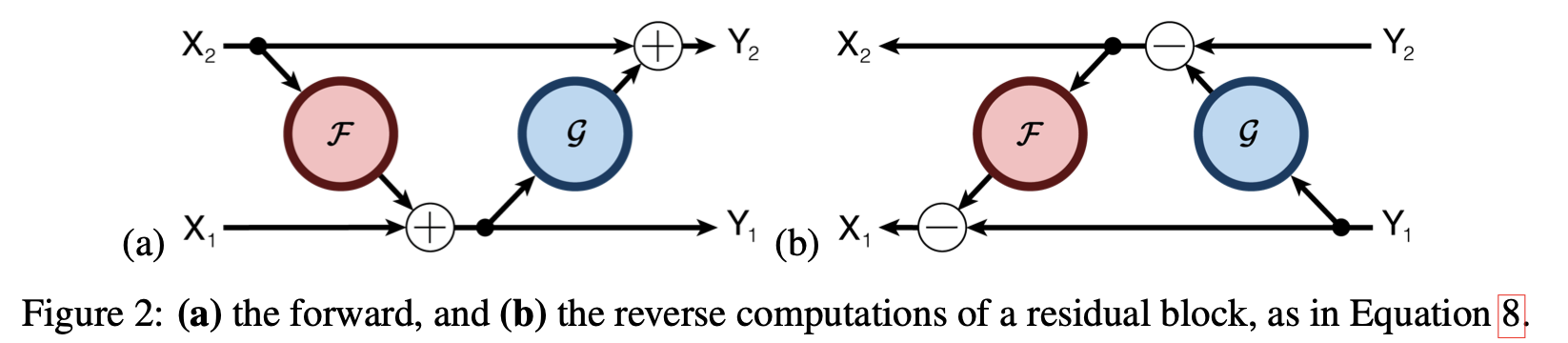The Reversible Residual Network: Backpropagation Without Storing Activations

The Reversible Residual Network (RevNet) idea is applied to a transformer, which is a Reformer, because the basic structure of a transformer is a repetition of Attention and FeedForward. Since the basic structure of a transformer is a repetition of Attention and FeedForward, $F, G$ in a transformer becomes Attention and FeedForward, and is shown as follows.

$$Y_1 = X_1 + Attention(X_2)$$

$$Y_2 = X_2 + FeedForward(X_1)$$

In addition, at the time of back program pagination, the

$$X_1 = Y_1 - Attention(X_2)$$

$$X_2 = Y_2 - FeedForward(X_1)$$

to find the value of the activation function after the activation of the previous layer.

Experiments have proven that the introduction of this calculation has no effect on accuracy. (Right side of Figure 3)

## Summary

Reformer ( ReversibleTransformer ), an attempt to reduce the computational complexity of transformers in natural language processing, was described. This enables training of more accurate models with fewer computational resources.

There are other models that attempt to reduce the computational complexity of the transformer, such as Longformer.

If you have any suggestions for improvement of the content of the article,